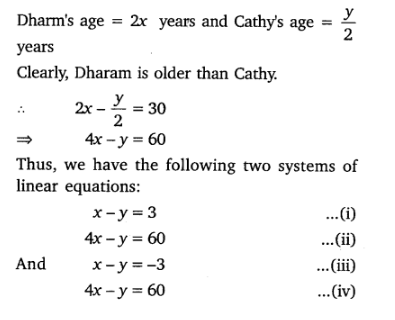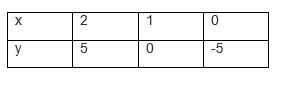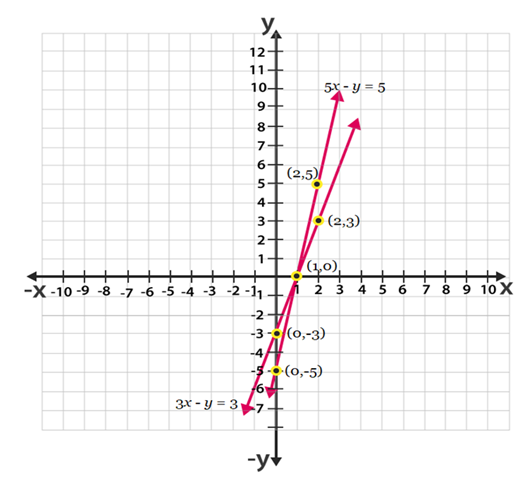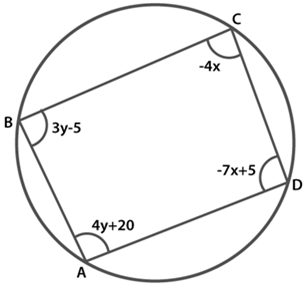• +91 9971497814
• info@interviewmaterial.com

# Pa.Linear Eq Ex-3.7 Interview Questions Answers

### Related Subjects

Question 1 : The age of two friends Ani and Biju differ by 3 years. Ani’s father Dharam is twice as old as Ani and Biju is twice as old as his sister Cathy. The ages of Cathy and Dharam differ by 30 years. Find the ages of Ani and Biju.

Let the ages of Ani and Biju be x years and y years respectively.
If Ani is older than Biju
x – y =3
If Biju is older than Ani
y – x = 3
-x + y =3   [Given]Subtracting equation (i) from equation (ii), we get:
3x – 57
⇒ x = 19
Putting x = 19 in equation (i), we get
19-y = 3
⇒ y = 16
Again subtracting equation (iv) from equation (iii), we get
3x = 63
⇒  x =  21
Putting x = 21 in equation (iii) we get
21 -y=  -3
⇒  y  =   24
Hence, Ani’s age is   either 19 years or 21 years and Biju’s age is either 16 years or 24 years.

Question 2 : One says, “Give me a hundred, friend! I shall then become twice as rich as you”. The other replies, “If you give me ten, I shall be six times as rich as you”. Tell me what is the amount of their (respective) capital?

Let the two friends have ₹ x and ₹ y.
According to the first condition:
One friend has an amount = ₹(x + 100)
Other has an amount = ₹ (y – 100
∴  (x + 100) =2 (y – 100)
⇒  x + 100 = 2y – 200
⇒ x – 2y = -300       …(i)
According to the second condition:
One friend has an amount = ₹(x – 10)
Other friend has an amount =₹ (y + 10)
∴  6(x – 10) = y + 10
⇒ 6x – 60 = y + 10
⇒    6x-y = 70                                        …(ii)
Multiplying (ii) equation by 2 and subtracting the result from equation (i), we get:
x – 12x = – 300 – 140
⇒ -11x = -440
⇒  x = 40
Substituting x = 40 in equation (ii), we get
6 x 40 – y = 70
⇒ -y   = 70- 24
⇒  y   = 170
Thus, the two friends have ₹ 40 and ₹ 170.

Question 3 : A train covered a certain distance at a uniform speed. If the train would have been 10 km/h faster, it would have taken 2 hours less than the scheduled time. And, if the train were slower by 10 km/h, it would have taken 3 hours more than the scheduled time. Find the distance covered by the train.

Let the original speed of the train be x km/h
and the time taken to complete the journey be y hours.            ‘
Then the distance covered = xy km
Case I: When speed = (x + 10) km/h and time taken = (y – 2) h
Distance = (x + 10) (y – 2) km
⇒   xy = (x + 10) (y – 2)
⇒ 10y – 2x = 20
⇒  5y – x = 10
⇒ -x + 5y = 10   …(i)

Case II: When speed = (x – 10) km/h and time taken = (y + 3) h
Distance = (x – 10) (y + 3) km
⇒  xy = (x – 10) (y + 3)
⇒ 3x- 10y = 30    …(ii)
Multiplying equation (i) by 3 and adding the result to equation (ii), we get
15y – 10y = 30 f 30
⇒ 5y = 60
⇒   y   = 12
Putting y = 12 in equation (ii), we get
3x- 10 x 12= 30
⇒  3x   = 150
⇒ x   = 50
∴  x = 50 and y =   12
Thus, original speed of train is 50 km/h and time taken by it is 12 h.
Distance covered by train = Speed x Time
=  50 x 12 = 600 km.

Question 4 : The students of a class are made to stand in rows. If 3 students are extra in a row, there would be 1 row less. If 3 students are less in a row, there would be 2 rows more. Find the number of students in the class.

Let the number of rows be A and the number of students in a row be B.
Total number of students = Number of rows x Number of students in a row
=AB
Using the information, that is given,
First Condition:
Total number of students = (A – 1) ( B + 3)
Or AB = ( A – 1 )(B + 3) = AB – B + 3A – 3
Or 3A – B – 3 = 0
Or 3A – Y = 3 – – – – – – – – – – – – – (1)
Second condition:
Total Number of students = (A + 2 ) ( B – 3 )
Or AB = AB + 2B – 3A – 6
Or 3A – 2B = -6 – – – – – – – – – (2)
When equation (2) is subtracted from (1)
(3A – B) – (3A – 2B) = 3 – (-6)
-B + 2B = 3 + 6B = 9
By using the equation (1) we get,
3A – 9 =3
3A = 9+3 = 12
A = 4
Number of rows, A = 4
Number of students in a row, B = 9
Number of total students in a class = AB = 4 x 9 = 36

Question 5 : In a ∆ABC, ∠C = 3 ∠B = 2(∠A + ∠B). Find the three angles.

Let ∠A = x° and ∠B = y°.
Then ∠C = 3∠B = (3y)°.
Now ∠A + ∠B + ∠C = 180°
⇒ x + y + 3y = 180°
⇒ x + 4y = 180° …(i)
Also, ∠C = 2(∠A + ∠B)
⇒ 3y – 2(x + y)
⇒ 2x – y = 0° …(ii)
Multiplying (ii) by 4 and adding the result to equation (i), we get:
9x = 180°
⇒ x = 20°
Putting x = 20 in equation (i), we get:
20 + 4y = 180°
⇒ 4y = 160°
⇒  y =  160/40  = 40°
∴ ∠A = 20°, ∠B = 40° and ∠C = 3 x 40° = 120°.

Question 6 : Draw the graphs of the equations 5x – y = 5 and 3x – y = 3. Determine the co-ordinates of the vertices of the triangle formed by these lines and the y axis.

Given,
5x – y = 5
=> y = 5x – 5
Its solution table will be.Also given,3x – y = 3
y = 3x – 3The graphical representation of these lines will be as follows:From the above graph we can see that the triangle formed is ∆ABC by the lines and the y axis. Also the coordinates of the vertices are A(1,0) ,  C(0,-5) and B(0,-3).

Question 7 : Solve the following pair of linear equations:

(i) px + qy = p – q
qx – py = p + q
(ii) ax + by = c
bx + ay = 1 + c
(iii) x/a – y/b = 0
ax + by = a2 + b2
(iv) (a – b)x + (a + b) y = a2 – 2ab – b2
(a + b)(x + y) = a2 + b2
(v) 152x – 378y = – 74
–378x + 152y = – 604

Solutions:

(i) px + qy = p – q……………(i)
qx – py = p + q……………….(ii)
Multiplying p to equation (1)  and q to equation (2), we get
p2x + pqy = p2 − pq ………… (iii)
q2x − pqy = pq + q2 ………… (iv)
Adding equation (iii) and equation (iv),we get
p2x + q2 x = p2  + q2
(p2 + q2 ) x = p2 + q2
x = (p2 + q2)/ p2 + q2 = 1
From equation (i), we get
p(1) + qy = p – q
qy = p-q-p
qy = -q
y = -1

(ii) ax + by= c…………………(i)
bx + ay = 1+ c………… ..(ii)
Multiplying a to equation (i) and  b to equation (ii), we obtain
a2x + aby = ac ………………… (iii)
b2x + aby = b + bc…………… (iv)
Subtracting equation (iv) from equation (iii),
(a2 – b2) x = ac − bc– b
x = (ac − bc– b)/ (a2 – b2)
x = c(a-b) –b / (a2+b2)
From equation (i), we obtain
ax +by = c
a{c(a−b)−b)/ (a2 – b2)} +by=c
ac(a−b)−ab/ (a2 – b2)+by=c
by=c–ac(a−b)−ab/(a2 – b2)
by=abc – b2 c+ab/a2-b2
y = c(a-b)+a/a2-b2

(iii) x/a – y/b = 0
ax + by = a2 + b2
x/a – y/b = 0
=> bx − ay = 0 ……. (i)
ax + by = a2 + b2 …….. (ii)
Multiplying a and b to equation (i) and (ii) respectively, we get
b2x − aby = 0 …………… (iii)
a2x + aby = a 3 + ab3 …… (iv)
Adding equations (iii) and (iv), we get
b2x + a2x = a 3 + ab2
x (b2 + a2) = a (a2 + b2) x = a
Using equation (i), we get
b(a) − ay = 0
ab − ay = 0
ay = ab,
y = b

(iv) (a – b)x + (a + b) y = a2 – 2ab – b2
(a + b)(x + y) = a2 + b2
(a + b) y + (a – b) x = a2− 2ab − b2 …………… (i)
(x + y)(a + b)  = a 2 + b2
(a + b) y + (a + b) x  = a2 + b2 ………………… (ii)
Subtracting equation (ii) from equation (i), we get
(a − b) x − (a + b) x = (a 2 − 2ab − b 2) − (a2 + b2)
x(a − b − a − b) = − 2ab − 2b2
− 2bx = − 2b (b + a)
x = b + a
Substituting this value in equation (i), we get
(a + b)(a − b)  +y (a + b)  = a2− 2ab – b2
a2 − b2 + y(a + b)  = a2− 2ab – b2
(a + b) y = − 2ab
y = -2ab/(a+b)

(v) 152x − 378y = − 74
76x − 189y = − 37
x =(189y-137)/76……………..…(i)
− 378x + 152y = − 604
− 189x + 76y = − 302 ………….. (ii)
Using the value of x in equation (ii), we get
−189(189y−37/76)+76y=−302
− (189)2y + 189 × 37 + (76)2 y = − 302 × 76
189 × 37 + 302 × 76 = (189)2 y − (76)2y
6993 + 22952 = (189 − 76) (189 + 76) y
29945 = (113) (265) y
y = 1
Using equation (i), we get
x = (189-37)/76
x = 152/76 = 2

Question 8 : ABCD is a cyclic quadrilateral (see Fig. 3.7). Find the angles of the cyclic quadrilateral.

Answer 8 :It is known that the sum of the opposite angles of a cyclic quadrilateral is 180o
Thus, we have
∠C +∠A = 180
4y + 20− 4x = 180
− 4x + 4y = 160
x − y = − 40 ……………(1)
And, ∠B + ∠D = 180
3y − 5 − 7x + 5 = 180
− 7x + 3y = 180 ………..(2)
Multiplying 3 to equation (1), we get
3x − 3y = − 120 ………(3)
Adding equation (2) to equation (3), we get
− 7x + 3x = 180 – 120
− 4x = 60
x = −15
Substituting this value in equation (i), we get
x − y = − 40
-y−15 = − 40
y = 40-15
= 25
∠A = 4y + 20 = 20+4(25) = 120°
∠B = 3y − 5 = − 5+3(25) = 70°
∠C = − 4x = − 4(− 15) = 60°
∠D = 5-7x
∠D= 5− 7(−15) = 110°
Hence, all the angles are measured.

Todays Deals### Pa.Linear Eq Ex-3.7 Contributorskrishan

Name:
Email:

# Latest News# 9000 interview questions in different categories